# The Gentlest Introduction to Tensorflow – Part 1

In this series of articles, we present the gentlest introduction to Tensorflow that starts off by showing how to do linear regression for a single feature problem, and expand from there.

By Soon Hin Khor, Co-organizer for Tokyo Tensorflow Meetup.

### Introduction

We are going to solve an overly simple, and unrealistic problem, which has the upside of making understanding the concepts of ML and TF easy. We want to predict a single scalar outcome, house price (in \$) based on a single feature, house size (in square meters, sqm). This eradicates the need to handle multi-dimensional data, enabling us to focus solely on defining a model, implementing, and training it in TF.

### Machine Learning (ML) In Brief

We start with a set of data points that we have collected (chart below), each representing the relationship between two values —an outcome (house price) and the influencing feature (house size).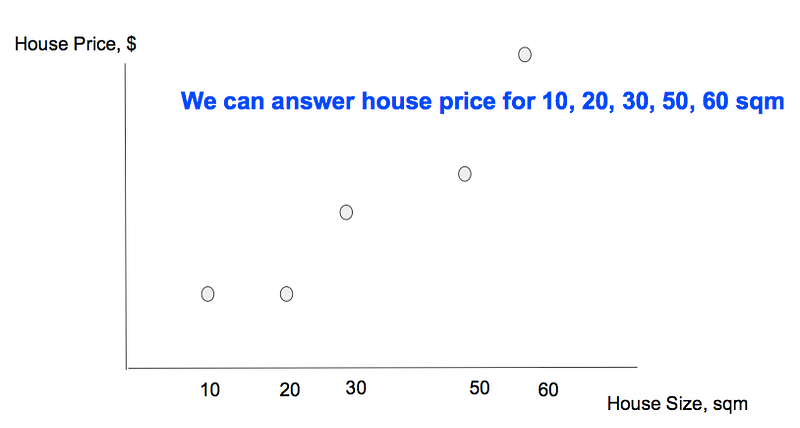However, we cannot predict values for features that we don’t have data points for (chart below)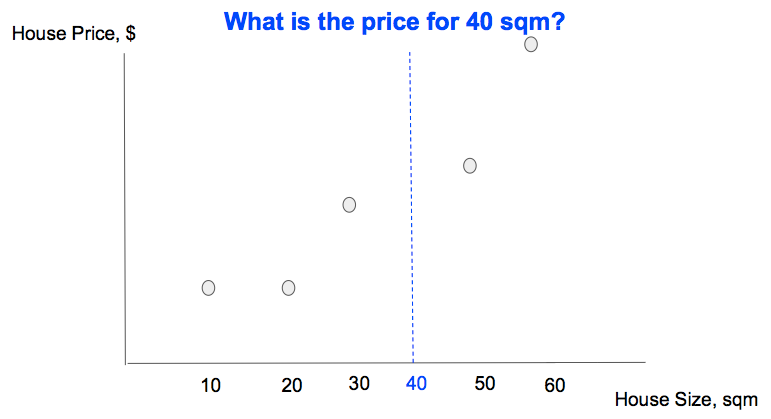We can use ML to discover the relationship (the ‘best-fit prediction line’ in the chart below), such that given a feature value that is not part of the data points, we can predict the outcome accurately (the intersection between the feature value and the prediction line.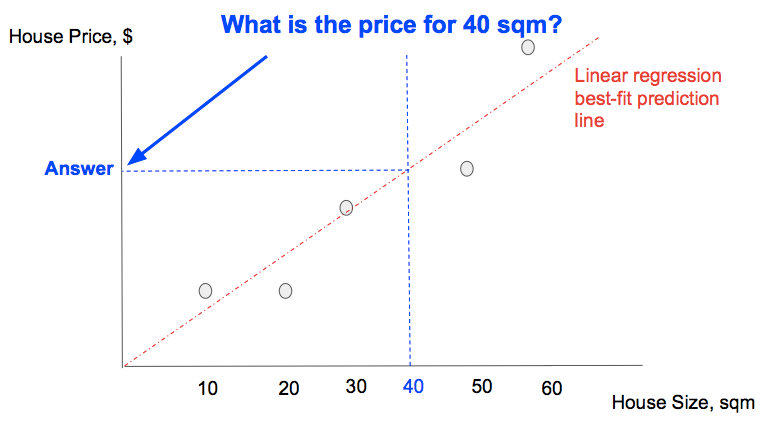### Step 1: Choose a Model

Model Types

To do prediction using ML, we need to choose a model that can best-fit the data that we have collected.

We can choose a linear (straight line) model, and tweak it to match the data points by changing its steepness/gradient and position.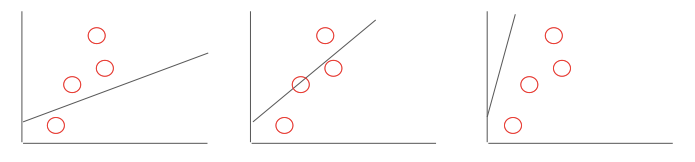We can also choose an exponential (curve) model, and tweak it to match the same set of data points by changing its curvature and position.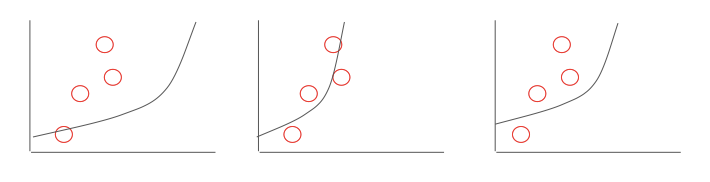Cost Function

To compare which model is a better-fit more rigorously, we define best-fit mathematically as a cost function that we need to minimize. An example of a cost function can simply be the absolute sum of the differences between the actual outcome represented by each data point, and the prediction of the outcome (the vertical projection of the actual outcome onto the best-fit line). Graphically the cost is depicted by the sum of the length of the blue lines in the chart below.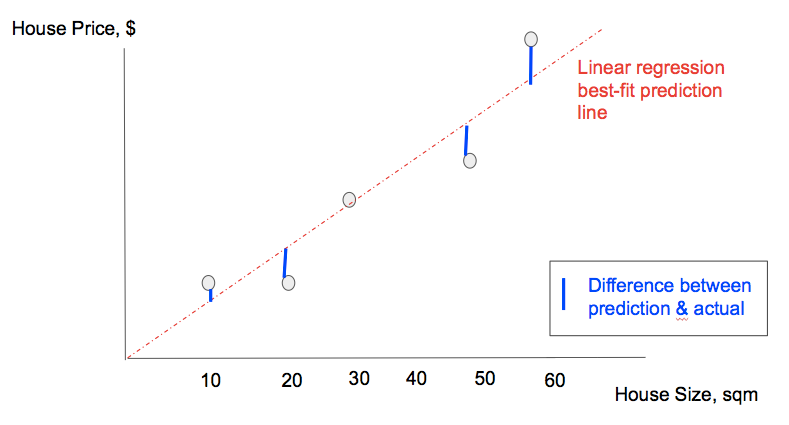NOTE: More accurately the cost function is often the squared of the difference between actual and predicted outcome, because the difference can sometimes can be negative; this is also known as min least-squared.

Linear Model In Brief

In the spirit of keeping things simple, we will model our data points using a linear model. A linear model is represented mathematically as:

```   y = W.x + b

Where:

x: house size, in sqm

y: predicted house price, in \$```

To tweak the model to best fit our data points, we can:

• Tweak W to change the gradient of the linear model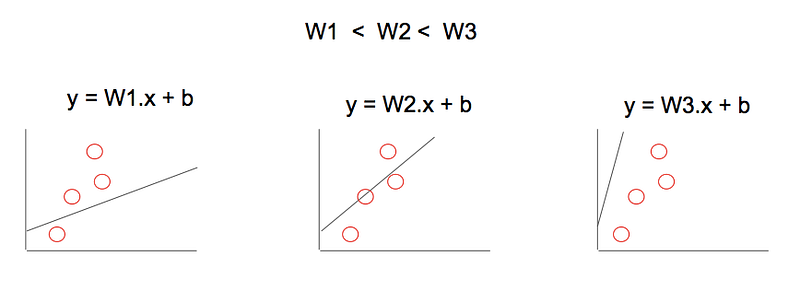• Tweak b to change the position of the linear model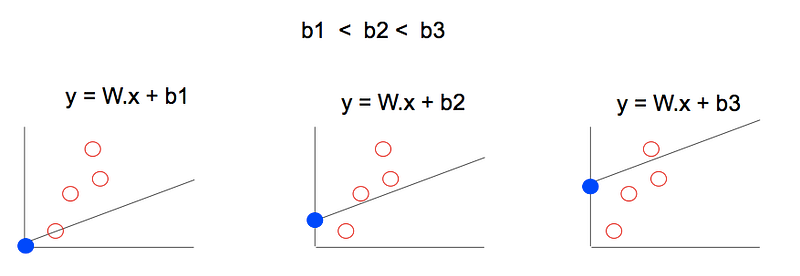By going through many values of W, b, we can eventually find a best-fit linear model that minimizes the cost function. Besides randomly trying different values, is there a better way to explore the W, b values quickly?

If you are on an expansive plateau in the mountains, when trying to descent to the lowest point, your viewpoint looks like this.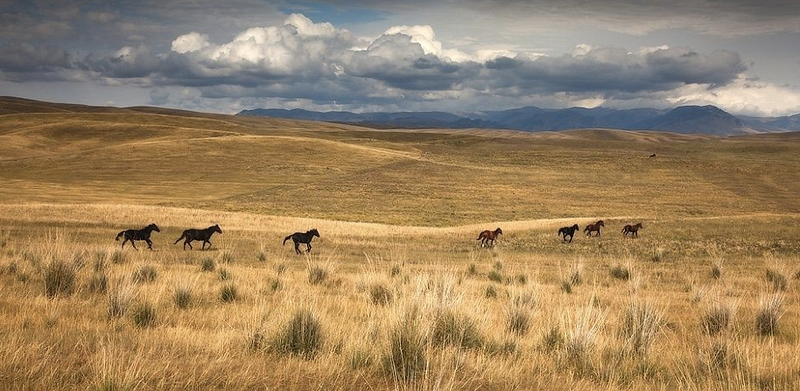The direction of descent is not obvious! The best way to descend is then to perform gradient descent:

• Determine the direction with the steepest downward gradient at current position
• Take a step of size X in that direction
• Repeat & rinse; this is known as training

Minimizing the cost function is similar because, the cost function is undulating like the mountains (chart below), and we are trying to find the minimum point, which we can similarly achieve through gradient descent.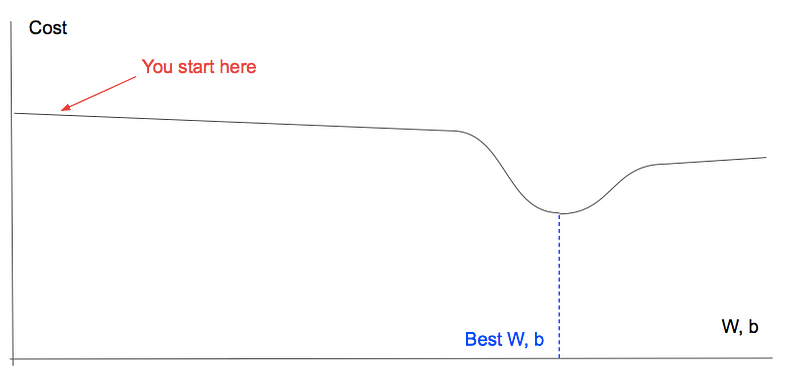With the concepts of linear model, cost function, and gradient descent in hand, we are ready to use TF.